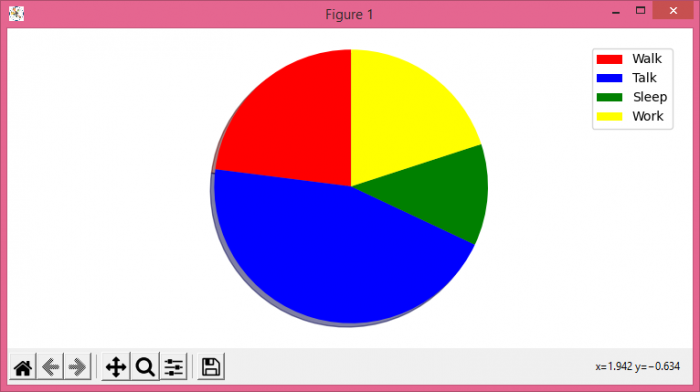# How to add a legend to a Matplotlib pie chart?

To add a legend to a Matplotlib pie chart, we can take the following steps −

• Set the figure size and adjust the padding between and around the subplots.
• Make a list of labels, colors, and sizes.
• Use pie() method to get patches and texts with colors and sizes.
• Place a legend on the plot with patches and labels.
• Set equal scaling (i.e., make circles circular) by changing the axis limits.
• To display the figure, use show() method.

## Example

import matplotlib.pyplot as plt

plt.rcParams["figure.figsize"] = [7.50, 3.50]
plt.rcParams["figure.autolayout"] = True

labels = ['Walk', 'Talk', 'Sleep', 'Work']
sizes = [23, 45, 12, 20]
colors = ['red', 'blue', 'green', 'yellow']

patches, texts = plt.pie(sizes, colors=colors, shadow=True, startangle=90)
plt.legend(patches, labels, loc="best")
plt.axis('equal')

plt.show()

## Output